# Formula Calc +

## Description

• This calculator can be calculated by inputting the formula unlike a usual calculator.
• The variable can be used in the formula. You can use alphabet(a-z) and greek alphabet(ā┐-āų) as the variable.
• You can edit formula with moving caret.
• You can create the graph by using the created formula.
• You can send the created formula with mail.
• You can solve some equations.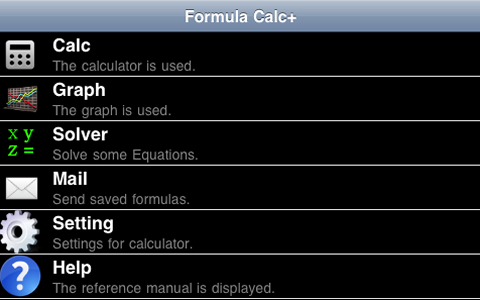Fig.1 Main Menu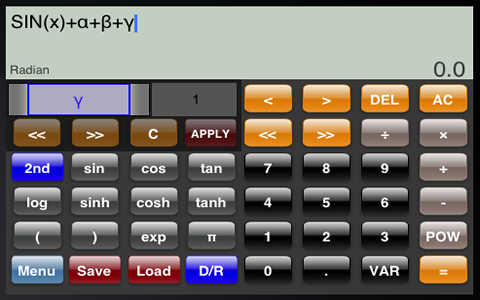Fig.2 Calcuraor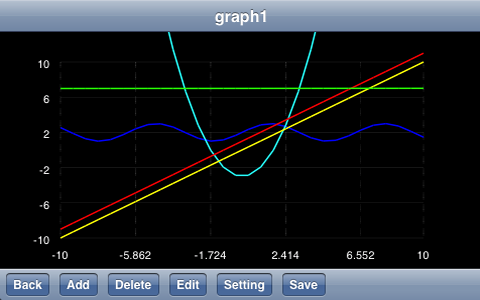Fig.3 Graph-Function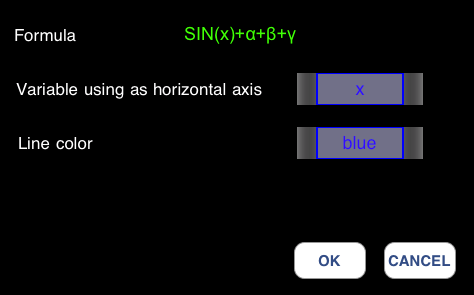Fig.4 You can select a variable as horizontal axis.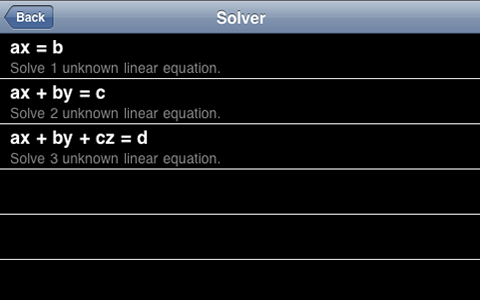Fig.5 Solver-Function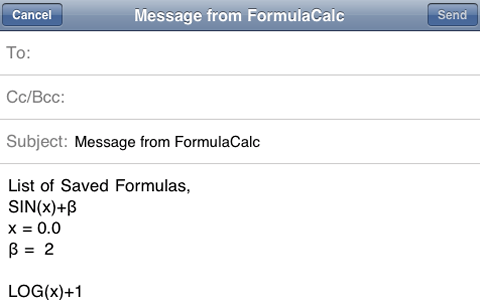Fig.6 Mail-Function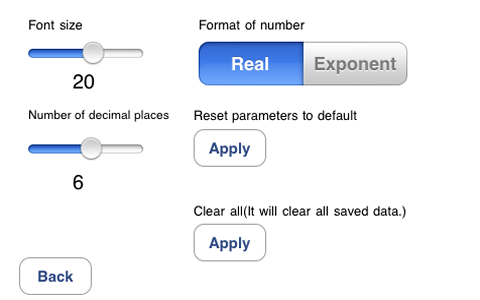Fig.7 Setting

Please refer to here if you want to know more detail.